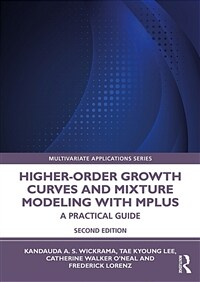> 상세정보

# 상세정보## Higher-order growth curves and mixture modeling with Mplus : a practical guide / 2nd ed

자료유형
단행본
개인저자
Wickrama, K. A. S., author
서명 / 저자사항
Higher-order growth curves and mixture modeling with Mplus : a practical guide / Kandauda A. S. Wickrama ... [et al.].
판사항
2nd ed.
발행사항
New York, NY :   Routledge,   2022.
형태사항
xviii, 326 p. : ill. ; 26 cm.
총서사항
Multivariate applications series
ISBN
9780367746209 9780367711269
요약
"This practical introduction to second-order and growth mixture models using Mplus introduces simple and complex techniques through incremental steps. The authors extend latent growth curves to second-order growth curve and mixture models and then combine the two using normal and non-normal (e.g., categorical) data. To maximize understanding, each model is presented with basic structural equations, figures with associated syntax that highlight what the statistics mean, Mplus applications, and an interpretation of results. Examples from a variety of disciplines demonstrate the use of the models and exercises allow readers to test their understanding of the techniques. A comprehensive introduction to confirmatory factor analysis, latent growth curve modeling, and growth mixture modeling is provided so the book can be used by readers of various skill levels. The book's datasets are available on the web. New to this edition: - Two new chapters providing a stepwise introduction and practical guide to the application of second-order growth curves and mixture models with categorical outcomes using Mplus program. Complete with exercises, answer keys, and downloadable data files. - Updated illustrative examples using Mplus 8.0 include conceptual figures, Mplus program syntax, and an interpretation of results to show readers how to carry out the analyses with actual data. This text is ideal for use in graduate courses or workshops on advanced structural equation, multilevel, longitudinal or latent variable modeling, latent growth curve and mixture modeling, factor analysis, multivariate statistics, or advanced quantitative techniques (methods) across the social and behaviorial sciences"--
서지주기
Includes bibliographical references and index.
일반주제명
Linear models (Statistics). Mathematical statistics.
 000 00000cam u22002058a 4500 001 000046111576 005 20220503132239 008 220331s2022 nyua b 001 0 eng 010 ▼a 2021025885 020 ▼a 9780367746209 ▼q (hardback) 020 ▼a 9780367711269 ▼q (paperback) 020 ▼z 9781003158769 ▼q (ebook) 035 ▼a (KERIS)REF000019629216 040 ▼a DLC ▼b eng ▼e rda ▼c DLC ▼d 211009 042 ▼a pcc 050 0 0 ▼a QA279 ▼b .W533 2022 082 0 0 ▼a 519.5 ▼2 23 084 ▼a 519.5 ▼2 DDCK 090 ▼a 519.5 ▼b H638-2 100 1 ▼a Wickrama, K. A. S., ▼e author ▼0 AUTH(211009)145512. 245 1 0 ▼a Higher-order growth curves and mixture modeling with Mplus : ▼b a practical guide / ▼c Kandauda A. S. Wickrama ... [et al.]. 250 ▼a 2nd ed. 260 ▼a New York, NY : ▼b Routledge, ▼c 2022. 264 1 ▼a New York, NY : ▼b Routledge, ▼c 2022. 300 ▼a xviii, 326 p. : ▼b ill. ; ▼c 26 cm. 336 ▼a text ▼b txt ▼2 rdacontent 337 ▼a unmediated ▼b n ▼2 rdamedia 338 ▼a volume ▼b nc ▼2 rdacarrier 490 1 ▼a Multivariate applications series 504 ▼a Includes bibliographical references and index. 520 ▼a "This practical introduction to second-order and growth mixture models using Mplus introduces simple and complex techniques through incremental steps. The authors extend latent growth curves to second-order growth curve and mixture models and then combine the two using normal and non-normal (e.g., categorical) data. To maximize understanding, each model is presented with basic structural equations, figures with associated syntax that highlight what the statistics mean, Mplus applications, and an interpretation of results. Examples from a variety of disciplines demonstrate the use of the models and exercises allow readers to test their understanding of the techniques. A comprehensive introduction to confirmatory factor analysis, latent growth curve modeling, and growth mixture modeling is provided so the book can be used by readers of various skill levels. The book's datasets are available on the web. New to this edition: - Two new chapters providing a stepwise introduction and practical guide to the application of second-order growth curves and mixture models with categorical outcomes using Mplus program. Complete with exercises, answer keys, and downloadable data files. - Updated illustrative examples using Mplus 8.0 include conceptual figures, Mplus program syntax, and an interpretation of results to show readers how to carry out the analyses with actual data. This text is ideal for use in graduate courses or workshops on advanced structural equation, multilevel, longitudinal or latent variable modeling, latent growth curve and mixture modeling, factor analysis, multivariate statistics, or advanced quantitative techniques (methods) across the social and behaviorial sciences"-- ▼c Provided by publisher. 630 0 0 ▼a Mplus. 650 0 ▼a Linear models (Statistics). 650 0 ▼a Mathematical statistics. 830 0 ▼a Multivariate applications series. 945 ▼a ITMT

### 소장정보

No. 소장처 청구기호 등록번호 도서상태 반납예정일 예약 서비스
No. 1 소장처 청구기호 519.5 H638-2 등록번호 111861516 도서상태 대출가능 반납예정일 예약 서비스

### 컨텐츠정보

#### 목차

```1 Introduction
2 Latent Growth Curves
3 Longitudinal Confirmatory Factor Analysis and Curve-of-Factors Growth Curve Models
4 Estimating Curve-of-Factors Growth Curve Models
5 Extending a Parallel Process Latent Growth Curve Model (PPM) to a Factor-of-Curves Model (FCM)
6 Estimating a Factor-of-Curves Model (FCM) and Adding Covariates
7 An Introduction to Growth Mixture Models (GMM)
8. Estimating a Conditional Growth Mixture Model (GMM)
9 Second-Order Growth Mixture Models (SOGMMs)
10. Growth Curve Analysis with Categorical Outcomes [NEW CHAPTER]
11. Higher-order Growth Curve Analysis with Categorical Outcomes [NEW CHAPTER]```

### 관련분야 신착자료

#### 학교에서 통계와 데이터과학은 어떻게 가르쳐야 할까? : 지도 및 평가를 위한 가이드라인

Bargagliotti, Anna (2022)

문건웅 (2022)

이상석 (2022)

강종경 (2022)

우종필 (2022)

김진현 (2022)

#### 경영경제 통계학

Doane, David P (2022)

#### 숫자에 속지 않고 숫자 읽는 법

Chivers, Tom (2022)

설현수 (2022)

민인식 (2022)

강상욱 (2022)

조진석 (2022)

#### 연구방법론 : 논문 시작부터 끝까지 SPSS / 2판

연구방법론 편찬위원회 (2022)

김은옥 (2022)

윤복식 (2022)

권세혁 (2022)

정성규 (2022)

민인식 (2022)